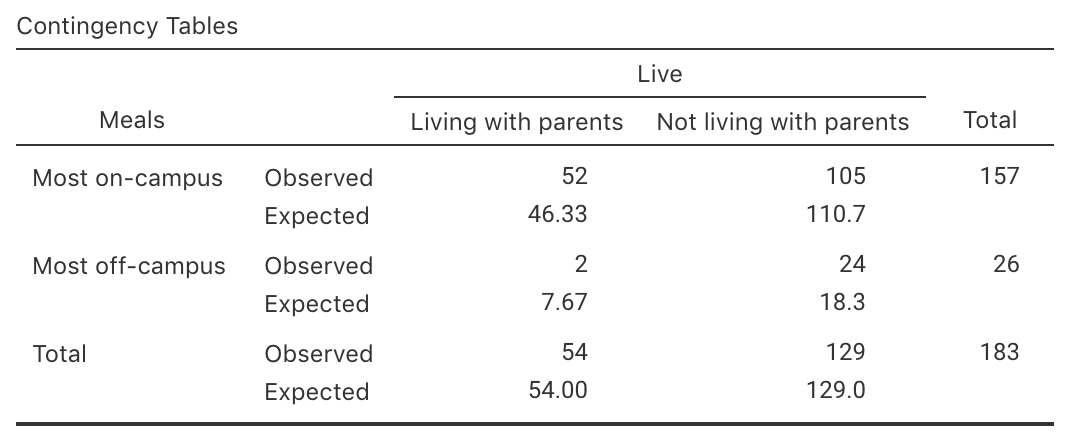## 31.7 Statistical validity conditions

As usual, these results hold under certain conditions. The test above is statistically valid if

• All expected counts are at least five.

Some books may give other (but similar) conditions.

In addition to the statistical validity condition, the test will be

The statistical validity condition refers to the expected (not the observed) counts. SPSS tells us if a problem exists with the expected count condition, underneath the first output table in Fig. 25.3. In jamovi, the expected counts must be explicitly requested to see if this condition is satisfied (Fig. 31.3).FIGURE 31.3: The expected values, as computed in jamovi

For the student-eating data, the smallest observed count is 2 (living with parents; most meals off-campus), but the smallest expected count is 7.67, which is greater than five. The size of the expected counts is important for the statistical validity condition.

Example 31.1 (Statistical validity) For the university-student eating data, all the cells have an expected count of at least five so the statistical validity condition is satisfied.